# Visualizations and Animations for Billiards

All plotting and animating for DynamicalBilliards.jl lies within a few well-defined functions from InteractiveDynamics.jl that use the Makie ecosystem.

This documentation page is built using versions:

using Pkg
Pkg.status(["DynamicalBilliards", "InteractiveDynamics"];
mode = PKGMODE_MANIFEST, io=stdout
)
      Status ~/work/InteractiveDynamics.jl/InteractiveDynamics.jl/docs/Manifest.toml
[4986ee89] DynamicalBilliards v4.0.0
[ec714cd0] InteractiveDynamics v0.21.4 ~/work/InteractiveDynamics.jl/InteractiveDynamics.jl

## Plotting

InteractiveDynamics.bdplotFunction
bdplot(x; kwargs...) → fig, ax
bdplot!(ax::Axis, x; kwargs...)

Plot an object x from DynamicalBilliards into a given axis (or a new figure). x can be an obstacle, a particle, a vector of particles, or a billiard.

bdplot!(ax,::Axis, o::Obstacle; kwargs...)

Keywords are propagated to lines! or poly!. Functions obfill, obcolor, obls, oblw (not exported) decide global defaults for linecolor, fillcolor, linestyle, linewidth, when plotting obstacles.

bdplot!(ax,::Axis, bd::Billiard; clean = true, kwargs...)

If clean = true, all axis elements are removed and an equal aspect ratio is establised. Other keywords are propagated to the obstacle plots.

bdplot!(ax,::Axis, bd::Billiard, xmin, xmax, ymin, ymax; kwargs...)

This call signature plots periodic billiards: it plots bd along its periodic vectors so that it fills the total amount of space specified by xmin, xmax, ymin, ymax.

bdplot!(ax,::Axis, ps::Vector{<:AbstractParticle}; kwargs...)

Plot particles as a scatter plot (positions) and a quiver plot (velocities). Keywords particle_size = 5, velocity_size = 0.05 set the size of plotted particles. Keyword colors = JULIADYNAMICS_CMAP decides the color of the particles, and can be either a colormap or a vector of colors with equal length to ps. The rest of the keywords are propagated to the scatter plot of the particles.

source
InteractiveDynamics.bdplot_boundarymapFunction
bdplot_boundarymap(bmap, intervals; figkwargs = NamedTuple(), kwargs...)

Plot the output of DynamicalBilliards.boundarymap into an axis that correctly displays information about obstacle arclengths.

Also works for the parallelized version of boundary map.

Keyword Arguments

• figkwargs = NamedTuple() keywords propagated to Figure.
• color : The color to use for the plotted points. Can be either a single color or a vector of colors of length length(bmap), in order to give each initial condition a different color (for parallelized version).
• All other keywords propagated to scatter!.
source

### Plotting an obstacle with keywords

using DynamicalBilliards, InteractiveDynamics, CairoMakie

bd = billiard_sinai()

fig, ax = bdplot(bd)
bdplot!(ax, bd; color = "blue", linestyle = :dot, linewidth = 5.0)
bdplot!(ax, bd; color = "yellow", strokecolor = "black")
fig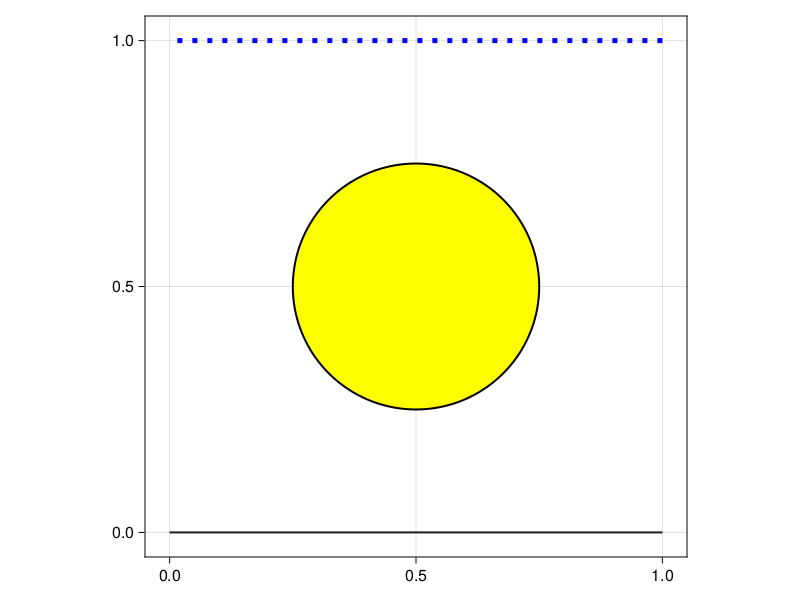### Plotting a billiard

using DynamicalBilliards, InteractiveDynamics, CairoMakie
bd = billiard_logo()
fig, ax = bdplot(bd)
fig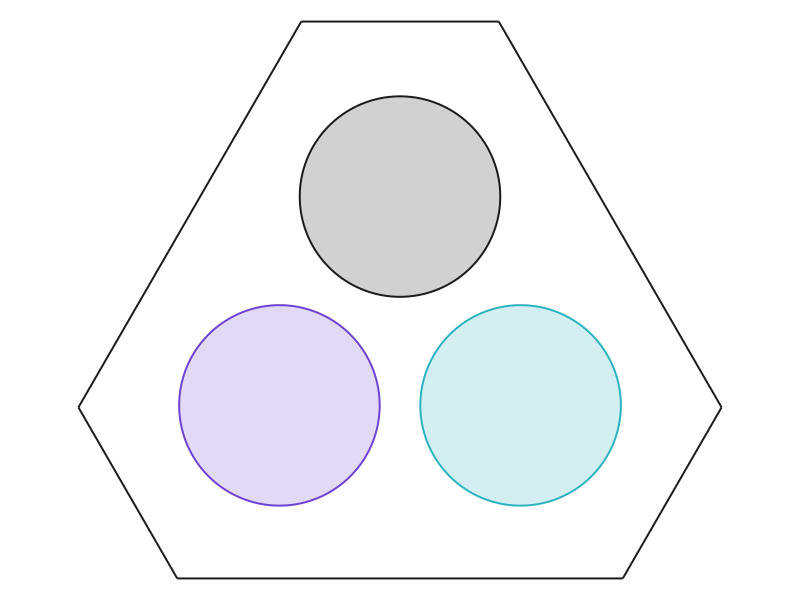### Plotting some particle trajectories

using DynamicalBilliards, InteractiveDynamics, CairoMakie

bd = billiard_hexagonal_sinai()
p1 = randominside(bd)
p2 = randominside(bd, 1.0)
markers = [:circle, :rect]
fig, ax = bdplot(bd)
for (p, c) in zip([p1, p2], colors)
x, y = DynamicalBilliards.timeseries!(p, bd, 20)
lines!(ax, x, y; color = c)
end
bdplot!(ax, [p1, p2]; colors, particle_size = 10, marker = markers)
fig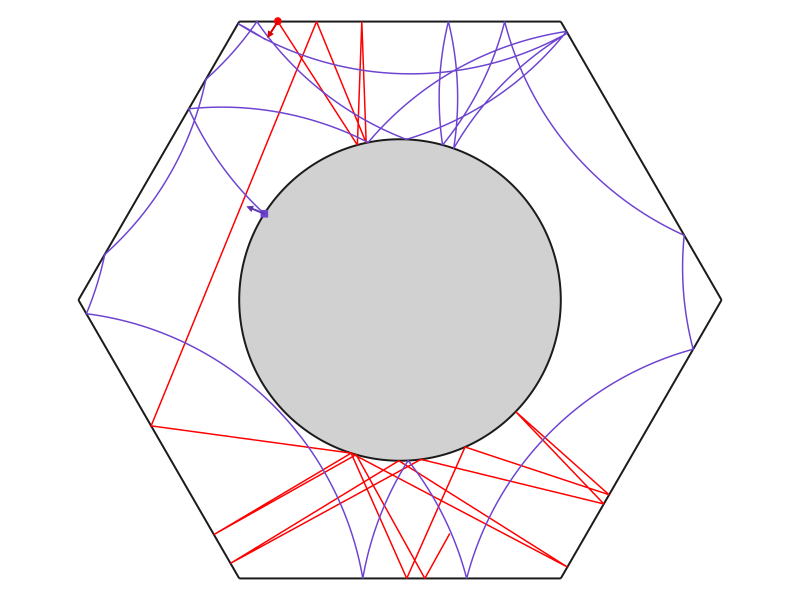### Periodic billiard plot

Rectangle periodicity:

using DynamicalBilliards, InteractiveDynamics, CairoMakie

r = 0.25
bd = billiard_rectangle(2, 1; setting = "periodic")
d = Disk([0.5, 0.5], r)
d2 = Ellipse([1.5, 0.5], 1.5r, 2r/3)
bd = Billiard(bd.obstacles..., d, d2)
p = Particle(1.0, 0.5, 0.1)
xt, yt, vxt, vyt, t = DynamicalBilliards.timeseries!(p, bd, 10)
fig, ax = bdplot(bd, extrema(xt)..., extrema(yt)...)
lines!(ax, xt, yt)
bdplot!(ax, p; velocity_size = 0.1)
fig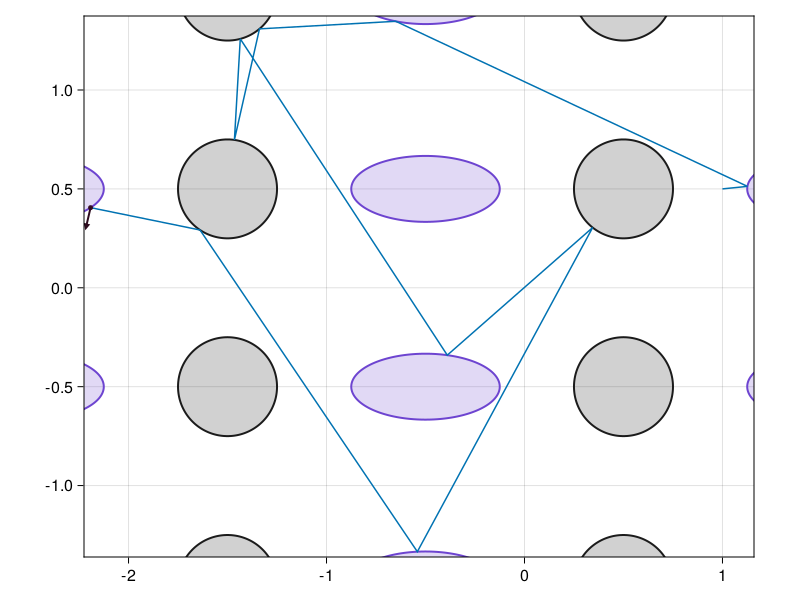Hexagonal periodicity:

using DynamicalBilliards, InteractiveDynamics, CairoMakie

bd = billiard_hexagonal_sinai(0.3, 1.50; setting = "periodic")
d = Disk([0.7, 0], 0.2)
d2 = Antidot([0.7/2, 0.65], 0.35)
bd = Billiard(bd..., d, d2)

p = MagneticParticle(-0.5, 0.5, π/5, 1.0)

xt, yt = DynamicalBilliards.timeseries(p, bd, 10)
fig, ax = bdplot(bd, extrema(xt)..., extrema(yt)...)
lines!(ax, xt, yt)
bdplot!(ax, p; velocity_size = 0.1)
fig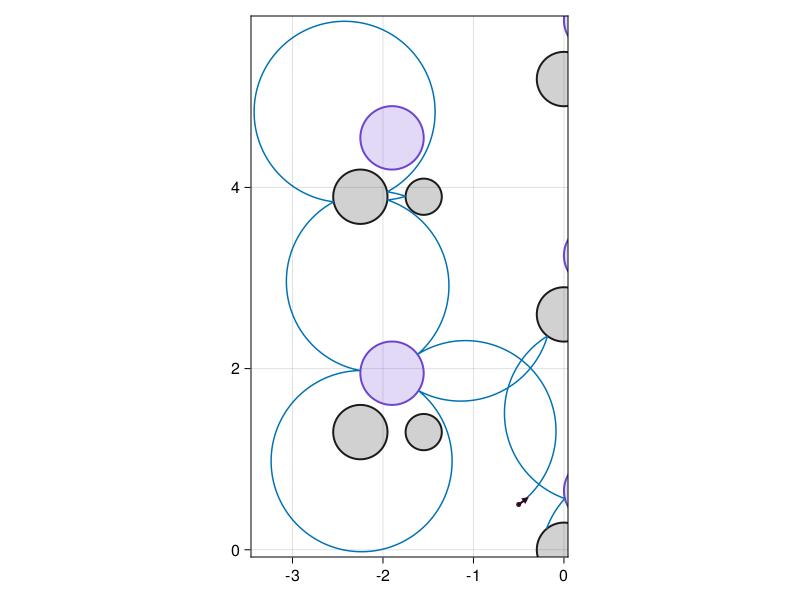### Boundary map plot

using DynamicalBilliards, InteractiveDynamics, CairoMakie

bd = billiard_mushroom()

n = 100 # how many particles to create
t = 200 # how long to evolve each one

bmap, arcs = parallelize(boundarymap, bd, t, n)

colors = [randomcolor() for i in 1:n] # random colors

fig, ax = bdplot_boundarymap(bmap, arcs, color = colors)
fig